National Aeronautics and Space Administration Glenn Research CenterEducational Product Teachers Grades 9-12

# V = I x R

Where: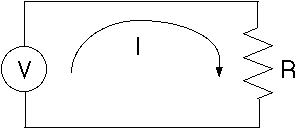V = Voltage

I = Current

R = Resistance

### Example Problem:

A nine volt battery supplies power to a cordless curling iron with a resistance of 18 ohms. How much current is flowing through the curling iron?

Sketch: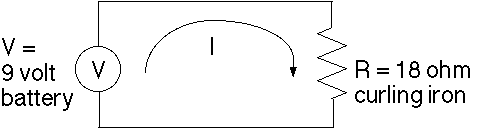### Solution: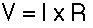1.) Since V(Voltage) and R(Resistance) are known, solve for I(Current) by dividing both sides of the equation by R.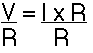2.) The R's on the right hand side of the equation cancel.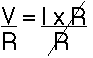3.) I is then left in terms of V and R.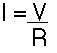4.) Substitute in the values for V(Voltage) and R(Resistance).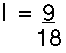5.) Solve for I(Current).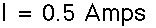### Problem #1

A 110 volt wall outlet supplies power to a strobe light with a resistance of 2200 ohms. How much current is flowing through the strobe light?

Sketch: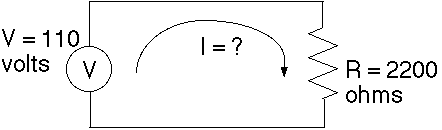Choose your answer below

## Problem #2

A CD player with a resistance of 40 ohms has a current of 0.1 amps flowing through it. Sketch the circuit diagram and calculate how many volts supply the CD player?

Choose your answer below

This activity was created by Steven Gutierrez, for the Learning Technologies Project, at the NASA Glenn Research Center, in 1996.

Ohm's Law Web Page

Navigation ..Beginner's Guide Home Page
Please direct any comments to:
Thomas.J.Benson@nasa.gov